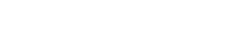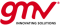If you wish to contribute or participate in the discussions about articles you are invited to contact the Editor

# Lock DetectorsReceivers
Title Lock Detectors
Edited by GMV
Year of Publication 2011In nominal situations, GNSS receivers continuously output a navigation solution using the measurements generated by the tracking loops. The receiver must therefore ensure that the tracking loops are correctly following the incoming signal, and that they have not diverged away from the solution. This is the purpose of the lock detectors, which produce quality factors to assess how well the signal is being tracked.

## Concept

The purpose of the lock detectors is to assess whether the incoming signal is being correctly tracked at channel level or not. For that purpose, the GNSS receiver evaluates pre-defined quality parameters in order to assess:

• Code lock of the DLL;
• Phase lock of the PLL;
• Frequency lock of the FLL;

These quality parameters may be obtained from the correlator outputs, or cross-checking of internal information, as detailed hereafter.

After computing the quality parameters, the receiver checks their values against pre-defined thresholds, which may depend on the application. As an example, if the receiver is targeting high accuracy, these thresholds might be more restrictive, whereas if the priority is solution availability, then these thresholds are more likely to be relaxed.

Once loss of lock is declared, the receiver may react in different ways. If only one of the loops loses lock (e.g. the PLL), then the receiver may decide to keep the other loops closed, and to restart only this loop. Conversely, the receiver may decide that this loss of lock is unrecoverable, and switches the channel back to acquisition (see also how receiver operations are done). These decisions are closely related to the receiver design, as they are often the result of all the performance trade-offs tailored to the target application.

## Code Lock Detectors

The concept behind the code lock detector is that if the DLL is working correctly, then the received signal power is high. Since the received signal is noisy, code lock is often assessed by comparing the estimated Carrier-to-Noise ratio, $\displaystyle{ \hat{C}/N_0\, }$, with a pre-defined threshold.

At the receiver, the $\displaystyle{ \hat{C}/N_0\, }$ can be computed using the ratio between signal plus noise power measured with different bandwidths. As such, the narrowband power computed over $\displaystyle{ M\, }$ and $\displaystyle{ k\, }$ integration periods can be written as:

$\displaystyle{ NBP_k=[\sum_M I_P]_k^2 + [\sum_M Q_P]_k^2\, }$

and the wide band power computed over $\displaystyle{ M\, }$ and $\displaystyle{ k\, }$ integration periods can be written as:

$\displaystyle{ WBP_k=[\sum_M (I_P^2 + Q_P^2)]_k\, }$

where:

• $\displaystyle{ I_P\, }$ and $\displaystyle{ Q_P\, }$ are the In-phase and Quadrature outputs of the prompt correlator, respectively (see correlators for further information).
• $\displaystyle{ M\, }$ is the number of coherent integrations for the lock detector.

The estimated mean of the narrow power is given by:

$\displaystyle{ \hat{\mu}_{NP}=\frac{1}{K}\sum_{k=1}^K \frac{NBP_k}{WBP_k}\, }$

where $\displaystyle{ K\, }$ is the number of non-coherent integrations for the lock detector.

Finally, the estimated Carrier-to-Noise ratio $\displaystyle{ \hat{C}/N_0\, }$ computed at the correlators is given by:

$\displaystyle{ \frac{\hat{C}}{N_0}=10 log_{10} (\frac{1}{T} \frac{\hat{\mu}_{NP} - 1}{M - \hat{\mu}_{NP}})\, }$

where $\displaystyle{ T\, }$ is the integration time.

## Phase Lock Detectors

The phase lock detector is based on the concept that, if the incoming signal is being correctly tracked, then the In-phase component I of the Prompt correlator is maximum, and its Quadrature component Q is minimum, as shown here.

A quality parameter often used at the receiver is the cosine of twice the phase of the prompt correlator, given by:

$\displaystyle{ cos(2\phi)=\frac{[\sum_M I_P]_k^2 - [\sum_M Q_P]_k^2}{[\sum_M I_P]_k^2 + [\sum_M Q_P]_k^2}\, }$

Note that, when in good lock conditions, this quality parameter is nearly 1.

## Frequency Lock Detectors

Frequency lock detectors are not so common in receiver implementations, since the receiver mainly relies on phase lock detectors and code lock detectors. In any case, one possible implementation of a frequency lock detector is to cross check that the carrier Doppler (velocity state) is consistent with the code Doppler (velocity state) measured at the DLL.